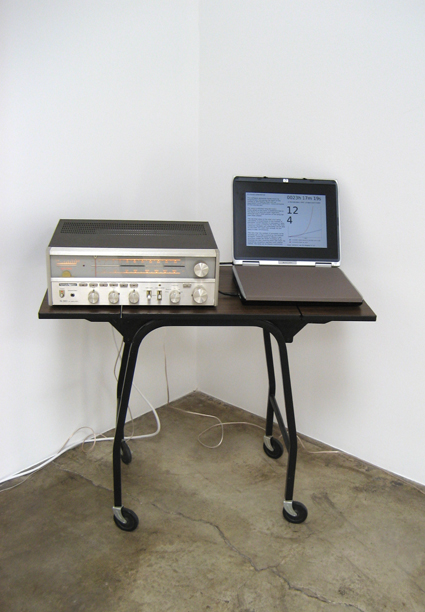Pi House Generator (2008) - Paul SlocumThis software randomly generates house music using the number pi. Pi is the ratio of a circle's diameter to its circumference, a number with infinite digits in a random non-repeating sequence.

The software progressively calculates the sequence of digits in pi, starting at 3.14 and progressing towards infinity. As the program calculates the digits, it feeds the results into an algorithmic music generator containing my structural criteria for house music. The resulting piece of house music is infinitely long and static and never repeats itself.

The number of processor cycles required to calculate pi increase with the number of digits it is calculated to. After months or years of playing the song, any fixed computer hardware will be unable to calculate the digits fast enough for the song to play continuously.

The rate that the number of processor cycles increase per pi-digit is bound by the formula N*log(N). However based on Moore's Law, processor power per dollar increases at an exponential rate, doubling every two years. By upgrading computers regularly with market trends, the song can be played indefinitely.

-- FROM THE ARTIST'S STATEMENT